Do you like the article?
Share it with others —
post a link to it!
Use new possibilities of MetaTrader 5

# Learn how to design a trading system by Relative Vigor Index

15 September 2022, 12:18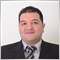0
7 122

### Introduction

Welcome to my new article in our series to learn new technical tools in detail and design a simple trading system with this tool. It is the Relative Vigor Index (RVI) indicator, we will cover it through the following topics:

We will use MQL5 (MetaQuotes Language 5) IDE to write our codes and the MetaTrader 5 trading terminal to execute our trading system. I advise you to try to apply what you will learn by yourself if you want to improve your coding skills as a beginner because practice is very important to do. If you do not know how to download and use MetaTrader 5 and MQL5, you can read the topic of Writing MQL5 code in MetaEditor from a previous article for me.

All mentioned strategies are for education purposes only, so, you may find that they need optimization and this is normal as the main objective of this article and these simple strategies is to learn the main concept behind it. So, you must test them very well before using them in your real account to make sure that they will be useful for your trading.

Disclaimer: All information provided 'as is' only for educational purposes and is not prepared for trading purposes or advice. The information does not guarantee any kind of result. If you choose to use these materials on any of your trading accounts, you will do that at your own risk and you will be the only responsible.

### Relative Vigor Index definition

In this topic, we will learn what is the Relative Vigor Index (RVI) in detail. It is a momentum and Oscillator technical indicator. By comparing the closing price to its opening level, this indicator measures the strength of the trend. During the uptrend, the closing price closes higher than the open price but during the downtrend, the closing price closes lower than the open price. In addition to that, the results were smoothed by using the simple moving average. This RVI indicator is performing better during trending markets than ranging periods according to its nature. We can find that the calculation of this RVI is similar to the Stochastic Oscillator but the RVI is comparing the closing price to its opening one and the Stochastic Oscillator is comparing the closing to its price range during a specific period. If we want to calculate the RVI manually, we can do that through the following steps:

RVI = (CLOSE - OPEN) / (HIGH - LOW)

Where:

CLOSE = Closing price

OPEN = Opening price

HIGH = Highest price

LOW = Lowest price

But usually, the RVI that we use appears as two lines with different components in calculation and the following is for how we can calculate them.

1- 1st line Calculation:

• Nominator calculation:

MovAverage = (CLOSE-OPEN) + 2 * (CLOSE-1 - OPEN-1) + 2 * (CLOSE-2 - OPEN-2) + (CLOSE-3 - OPEN-3)

Where:

CLOSE = Current closing price

CLOSE 1, CLOSE 2, CLOSE 3 = Closing prices of 1, 2, and 3 periods ago

OPEN = Current opening price

OPEN 1, OPEN 2, OPEN 3 = Opening prices of 1, 2, and 3 periods ago

• Denominator calculation:

RangeAverage = (HIGH-LOW) + 2 x (HIGH-1 - LOW-1) + 2 x (HIGH-2 - LOW-2) + (HIGH-3 - LOW-3)

Where:

HIGH = Last Maximal Price

HIGH, HIGH 2, HIGH 3 = Last Maximal Prices of 1, 2, and 3 periods ago

LOW = Last Minimal Price

LOW 1, LOW 2, LOW 3 = Last Minimal Prices of 1, 2, and 3 periods ago

• Calculate the sum of these averages the same as the following: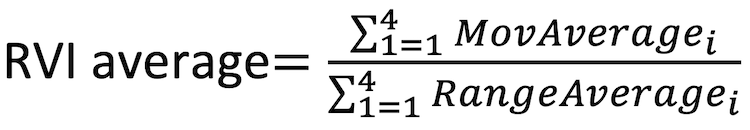2- 2nd line calculation:

RVIsignal = (RVIaverage + 2 * RVIaverage-1 + 2 * RVIaverage-2 + RVIaverage-3)/6

Now, we understood the main concept behind this indicator after learning how to calculate it manually but we do not need to do that because it is calculated in the MetaTrader 5 trading terminal and all that we need to do is to choose it from the available indicators. The following is for how to insert it into the chart:

While opening the MetaTrader 5 terminal --> Insert --> Indicators --> Oscillators --> Relative Vigor Index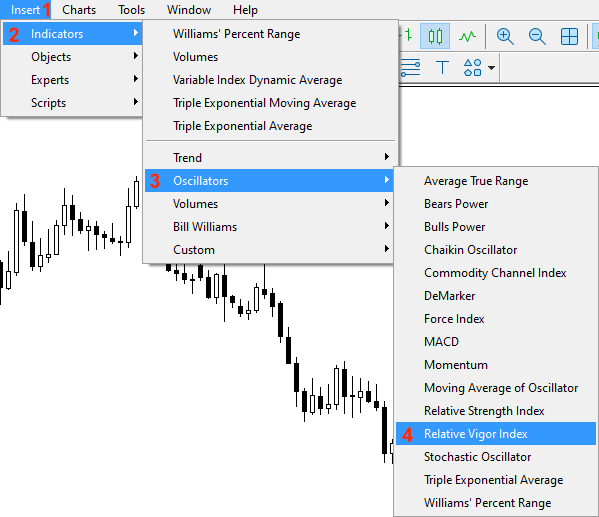After pressing on the Relative Vigor Index, we will see the window of its parameters the same as the following: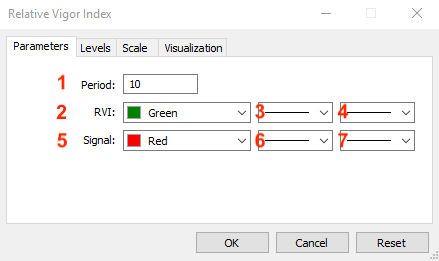1- To determine the period.

2- To determine the color of the RVI line.

3- To determine the style of the RVI line.

4- To determine the thickness of the RVI line.

5- To determine the color of the signal line.

6- To determine the style of the signal line.

7- To determine the thickness of the signal line.

After determining all of the parameters, we will find that the indicator is attached the same as the following: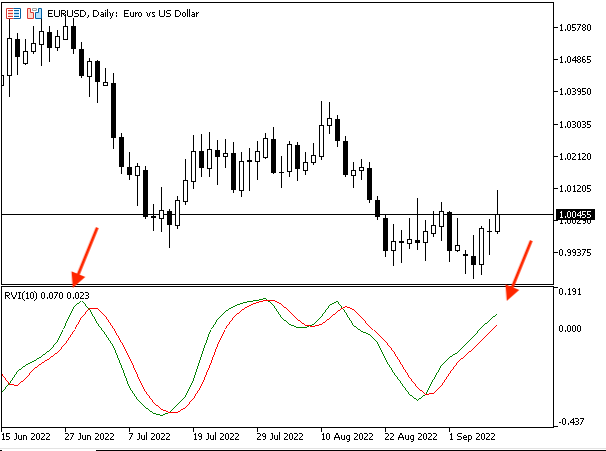As we can see in the previous chart that we have the RVI indicator is attached to the chart and it has two lines oscillating around the zero level.

### Relative Vigor Index strategy

After learning the main concept behind the RVI, we will learn how to use it based on its main concept through simple strategies. What I need to confirm here again is to make sure to test any strategy before using it on your real account to make sure that it is suitable for your trading and profitable as the main concept here is to share simple strategies for educational purposes only and it is normal that you find that they may need optimizations.

• Strategy one: RVI Crossover - Uptrend:

Based on this strategy, we need to get buy and close signals during the uptrend by a specific condition. When the RVI current value and RVI signal current value are greater than the zero level at the same time that RVI current value is greater than the current value of the RVI signal, this will be a buy signal. Vice Versa, when the RVI current value and RVI signal current value is below zero level at the same time that the RVI current value is below the current value of the RVI signal, this will be a close signal.

Simply,

RVI value > 0 and RVI signal value > 0 and RVI value > RVI signal value --> buy

RVI value < 0 and RVI signal value < 0 and RVI value < RVI signal value --> close

• Strategy two: RVI Crossover - Downtrend:

Based on this strategy, we need to get the opposite signals of the previous RVI Crossover - Uptrend strategy as we need to get short and cover signals. When the RVI current value and RVI signal current value are lower than the zero level at the same time that RVI current value is lower than the current value of the RVI signal, this will be a short signal. Vice Versa, when the RVI current value and RVI signal current value is above the zero level at the same time that the RVI current value is above the current value of the RVI signal, this will be a cover signal.

Simply,

RVI value < 0 and RVI signal value < 0 and RVI value < RVI signal value --> short

RVI value > 0 and RVI signal value > 0 and RVI value > RVI signal value --> cover

• Strategy three: RVI and MA Crossover:

Based on this strategy, we need to get buy and sell signals based on specific conditions as we need to get a buy signal when the closing price is greater than the 100 -period moving average at the same time that the current RVI value is greater than the current RVI signal value. In the other scenario, we need to get a sell signal when the closing price is lower than the 100 -period moving average at the same time that the current RVI value is lower than the current RVI signal value.

Simply,

Closing price >100- Moving average and RVI value > RVI signal value --> buy

Closing price <100- Moving average and RVI value < RVI signal value --> sell

### Relative Vigor Index strategy blueprint

After learning how we can use the RVI through our mentioned strategy, we will create a step-by-step blueprint for each strategy to help us to create a trading system smoothly.

• Strategy one: RVI Crossover - Uptrend:

According to this strategy, we need to create a trading system that gives buy and close signals during the uptrend by continuously checking the following values:

• The current RVI value
• The current RVI signal value
• The zero level of the RVI indicator

If the current RVI value is greater than the zero level, the current RVI signal value is greater than the zero level, and the current RVI value is greater than the current RVI signal value, we need the trading system to return the following values as a comment on the chart:

• Relative Vigor Index Value
• RVI Signal Value

In the other scenario, if the current RVI value is lower than the zero level, the current RVI signal value is lower than the zero level, and the current RVI value is lower than the current RVI signal value, we need the trading system to return the following values as a comment on the chart:

• Close Signal
• Relative Vigor Index Value
• RVI Signal Value

The following is the step-by-step blueprint for this strategy: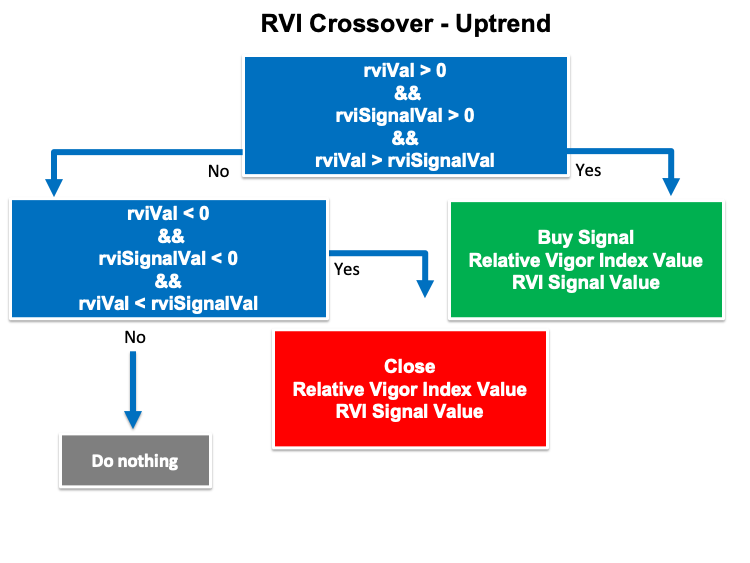• Strategy two: RVI Crossover - Downtrend:

According to this strategy, we need to create a trading system that can give us signals of short and cover during the downtrend by continuously checking the same values of the previous strategy:

• The current RVI value
• The current RVI signal value
• The zero level of the RVI indicator

But conditions will be slightly different as per the desired signals. If the current RVI value is lower than the zero level, the current RVI signal value is lower than the zero level at the same time, the RVI value is lower than the RVI signal value, we need the trading system to return the following values as a comment on the chart:

• Short Signal
• Relative Vigor Index Value
• RVI Signal Value

On the other hand, if the current RVI value is higher than the zero level, the current RVI signal value is higher than the zero level at the same time, the RVI value is higher than the RVI signal value, we need the trading system to return the following values as a comment on the chart:

• Cover
• Relative Vigor Index Value
• RVI Signal Value

The following is the blueprint of this trading system: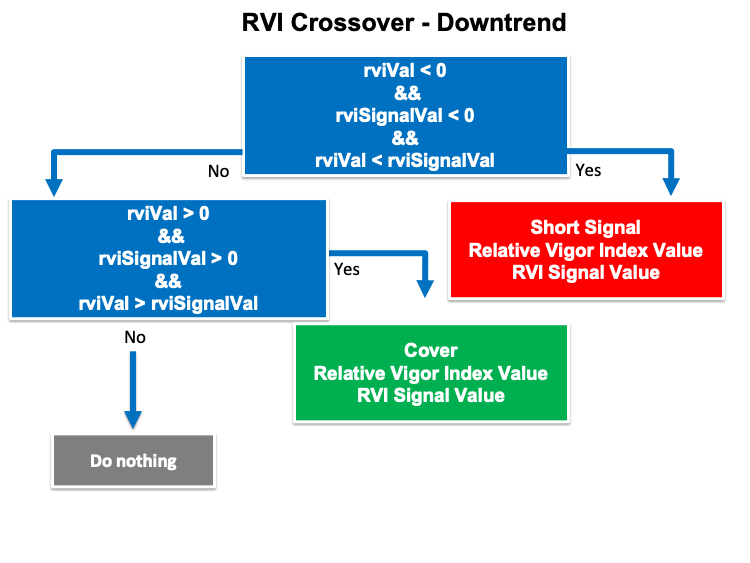• Strategy three: RVI & MA Crossover

According to this strategy, we need to create a trading system that gives buy and sell signals by continuously checking the following values:

• The closing price
• The 100- period moving average (MA)
• The current RVI value
• The current RVI signal value

If the closing price is higher than the 100- period MA and the current RVI value is higher than the current RVI signal value, we need the trading system to return the following values as a comment on the chart:

• Closing price
• MA Value
• Relative Vigor Index Value
• RVI Signal Value

On the other hand,  If the closing price is lower than the 100- period MA and the current RVI value is lower than the current RVI signal value, we need the trading system to return the following values as a comment on the chart:

• Sell Signal
• Closing price
• MA Value
• Relative Vigor Index Value
• RVI Signal Value

The following is the blueprint of this strategy: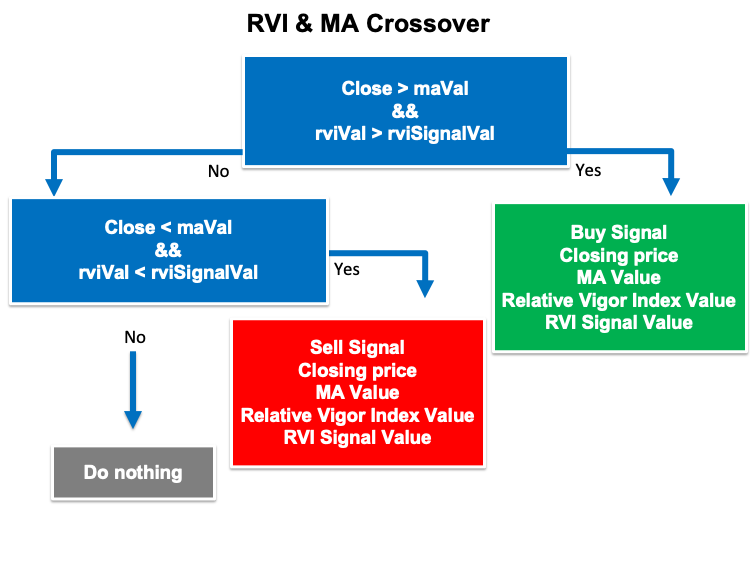### Relative Vigor Index trading system

After learning how to use the RVI through simple strategies and creating a step-by-step blueprint for each strategy to help us to create our trading system. Now, we will create a trading system for each mentioned strategy. First, we will create a simple trading system that returns the current RVI value as a comment on the chart and the following is for the code to create this trading system step by step.

Creating two arrays for the RVI and RVI signal by using the double function:

```   double rviArray[];
double rviSignalArray[];```

Setting the AS_SERIES flag to arrays to return a true or false which is a boolean value by using the "ArraySetAsSeries" function:

```   ArraySetAsSeries(rviArray,true);
ArraySetAsSeries(rviSignalArray,true);```

Defining the RVI by using the "iRVI" function that returns the handle of the Relative Vigor Index indicator. Its parameters are:

• symbol: to determine the symbol name, we will use (_Symbol) to be applied to the current symbol.
• period: to set the useable period, we will use (_period) to be applied to the current timeframe.
•  ma_period: to set the averaging period, we will use(10).
`int rviDef = iRVI(_Symbol,_Period,10);`

Getting data from the buffer of the RVI indicator by using the "CopyBuffer" function. Its parameters are:

• indicator_handle: we will use the (rviDef, rviDef) of the predefined indicator handle.
• buffer_num: to determine the indicator buffer number, we will use (0, 1).
• start_pos: to determine the starting position, we will use (0, 0).
• count: to determine the amount to copy, we will use (3, 3).
• buffer[]: to determine the target array to copy, we will use (rviArray, rviSignalArray).
```   CopyBuffer(rviDef,0,0,3,rviArray);
CopyBuffer(rviDef,1,0,3,rviSignalArray);```

Defining the values of RVI and RVI signal by using the "NormalizeDouble" function to return a value of double type. Its parameters are:

• value: to determine the normalized number. We will use (rviArray, rviSignalArray).
• digits: to determine the number of digits after the decimal. We will use (3).
```   double rviVal = NormalizeDouble(rviArray,3);
double rviSignalVal = NormalizeDouble(rviSignalArray,3);```

Using the "comment" function to return the value of the current RVI indicator as a comment on the chart.

```   Comment("Relative Vigor Index Value is ",rviVal,"\n",
"RVI Signal Value is ",rviSignalVal);```

The full code will be the same as the following:

```//+------------------------------------------------------------------+
//|                                                   Simple RVI.mq5 |
//|                                  Copyright 2022, MetaQuotes Ltd. |
//|                                             https://www.mql5.com |
//+------------------------------------------------------------------+
#property version   "1.00"
//+------------------------------------------------------------------+
void OnTick()
{
double rviArray[];
double rviSignalArray[];

ArraySetAsSeries(rviArray,true);
ArraySetAsSeries(rviSignalArray,true);

int rviDef = iRVI(_Symbol,_Period,10);

CopyBuffer(rviDef,0,0,3,rviArray);
CopyBuffer(rviDef,1,0,3,rviSignalArray);

double rviVal = NormalizeDouble(rviArray,3);
double rviSignalVal = NormalizeDouble(rviSignalArray,3);

Comment("Relative Vigor Index Value is ",rviVal,"\n",
"RVI Signal Value is ",rviSignalVal);

}
//+------------------------------------------------------------------+
```

After compiling this code, we will find the expert in the navigator window the same as the following: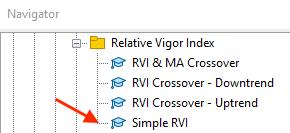By double-clicking the expert to execute it in the trading terminal, we will find the window of this expert the same as the following: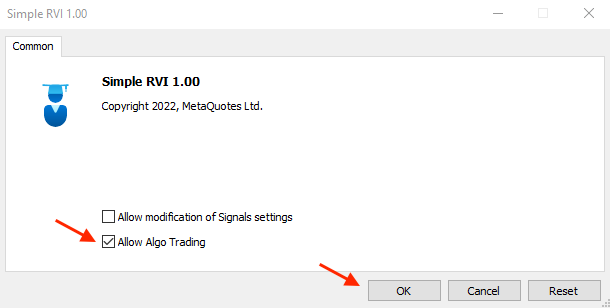By pressing "OK" after ticking next to the "Allow Algo Trading", we will find the expert is attached t the chart the same as the following: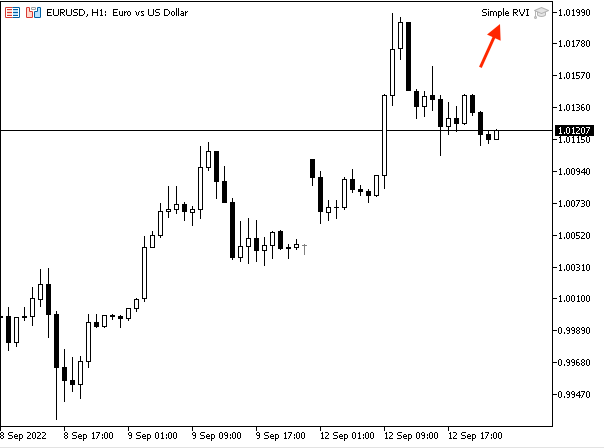Now, we're ready to get generated signals based on this trading system. So, we will get a signal the same as the following: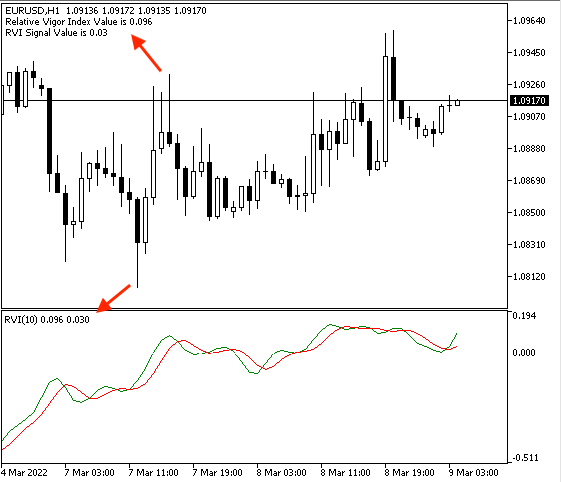As we can see on the top left corner of the chart we have a comment with the current RVI value.

• Strategy one: RVI Crossover - Uptrend:

The following is the full code to create a trading system based on this strategy:

```//+------------------------------------------------------------------+
//|                                      RVI Crossover - Uptrend.mq5 |
//|                                  Copyright 2022, MetaQuotes Ltd. |
//|                                             https://www.mql5.com |
//+------------------------------------------------------------------+
#property version   "1.00"
//+------------------------------------------------------------------+
void OnTick()
{
double rviArray[];
double rviSignalArray[];

ArraySetAsSeries(rviArray,true);
ArraySetAsSeries(rviSignalArray,true);

int rviDef = iRVI(_Symbol,_Period,10);

CopyBuffer(rviDef,0,0,3,rviArray);
CopyBuffer(rviDef,1,0,3,rviSignalArray);

double rviVal = NormalizeDouble(rviArray,3);
double rviSignalVal = NormalizeDouble(rviSignalArray,3);

if(rviVal>0 && rviSignalVal>0 && rviVal>rviSignalVal)
{
"Relative Vigor Index Value is ",rviVal,"\n",
"RVI Signal Value is ",rviSignalVal);
}
if(rviVal<0 && rviSignalVal<0 && rviVal<rviSignalVal)
{
Comment("Close","\n",
"Relative Vigor Index Value is ",rviVal,"\n",
"RVI Signal Value is ",rviSignalVal);
}

}
//+------------------------------------------------------------------+
```

Differences in this code.

Conditions of the strategy:

In case of buy signal

```   if(rviVal>0 && rviSignalVal>0 && rviVal>rviSignalVal)
{
"Relative Vigor Index Value is ",rviVal,"\n",
"RVI Signal Value is ",rviSignalVal);
}```

In case of close

```   if(rviVal<0 && rviSignalVal<0 && rviVal<rviSignalVal)
{
Comment("Close","\n",
"Relative Vigor Index Value is ",rviVal,"\n",
"RVI Signal Value is ",rviSignalVal);
}```

After compiling this code and executing it to the trading terminal in the same way as what we mentioned in the simple RVI trading system, we will find the expert is attached to the chart the same as the following: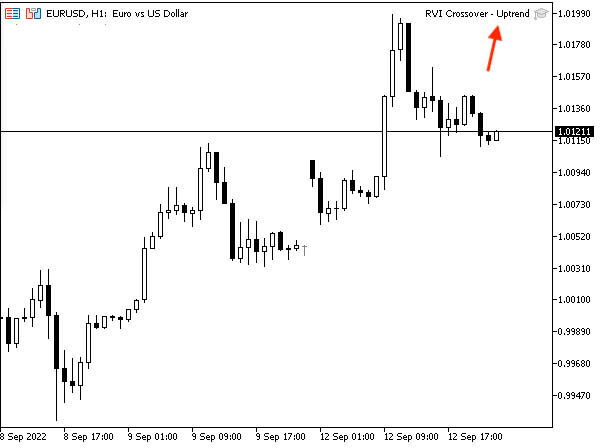Then, we can get desired signals based on this trading system the same as the following:

In case of buy signal: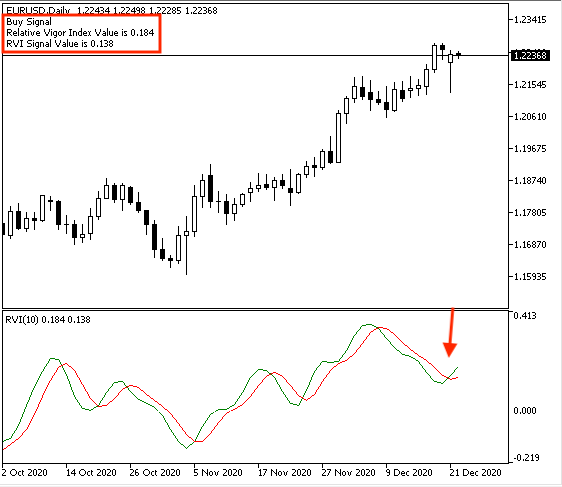As we can see on the chart in the top left corner that we received a signal with the following values:

• Relative Vigor Index value
• RVI signal value

In case of close signal: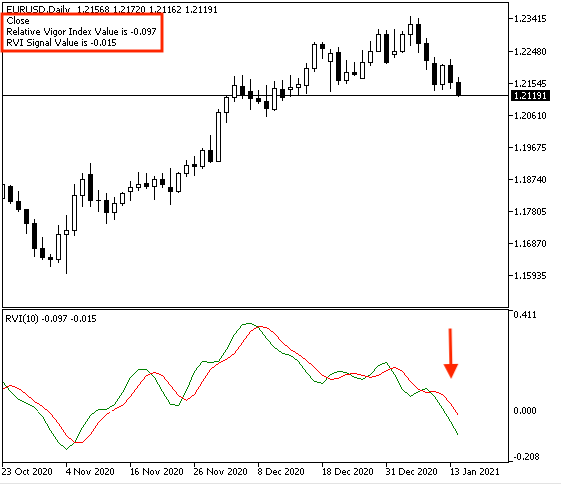As we can see on the chart in the top left corner that we received a signal with the following values:

• Close
• Relative Vigor Index value
• RVI signal value

It is clear that this signal is the same as what we need based on our mentioned trading strategy and its conditions during the uptrend.

• Strategy two: RVI Crossover - Downtrend:

The following is the full code to create a trading system for this trading strategy:

```//+------------------------------------------------------------------+
//|                                    RVI Crossover - Downtrend.mq5 |
//|                                  Copyright 2022, MetaQuotes Ltd. |
//|                                             https://www.mql5.com |
//+------------------------------------------------------------------+
#property version   "1.00"
//+------------------------------------------------------------------+
void OnTick()
{
double rviArray[];
double rviSignalArray[];

ArraySetAsSeries(rviArray,true);
ArraySetAsSeries(rviSignalArray,true);

int rviDef = iRVI(_Symbol,_Period,10);

CopyBuffer(rviDef,0,0,3,rviArray);
CopyBuffer(rviDef,1,0,3,rviSignalArray);

double rviVal = NormalizeDouble(rviArray,3);
double rviSignalVal = NormalizeDouble(rviSignalArray,3);

if(rviVal<0 && rviSignalVal<0 && rviVal<rviSignalVal)
{
Comment("Short Signal","\n",
"Relative Vigor Index Value is ",rviVal,"\n",
"RVI Signal Value is ",rviSignalVal);
}
if(rviVal>0 && rviSignalVal>0 && rviVal>rviSignalVal)
{
Comment("Cover","\n",
"Relative Vigor Index Value is ",rviVal,"\n",
"RVI Signal Value is ",rviSignalVal);
}
}
//+------------------------------------------------------------------+  ```

Differences in this code.

Conditions of the strategy:

In case of short signal

```   if(rviVal<0 && rviSignalVal<0 && rviVal<rviSignalVal)
{
Comment("Short Signal","\n",
"Relative Vigor Index Value is ",rviVal,"\n",
"RVI Signal Value is ",rviSignalVal);
}```

In case of cover

```   if(rviVal>0 && rviSignalVal>0 && rviVal>rviSignalVal)
{
Comment("Cover","\n",
"Relative Vigor Index Value is ",rviVal,"\n",
"RVI Signal Value is ",rviSignalVal);
}```

After compiling and executing this code the same as what we learned before, we will find that the expert is attached to the chart the same as the following: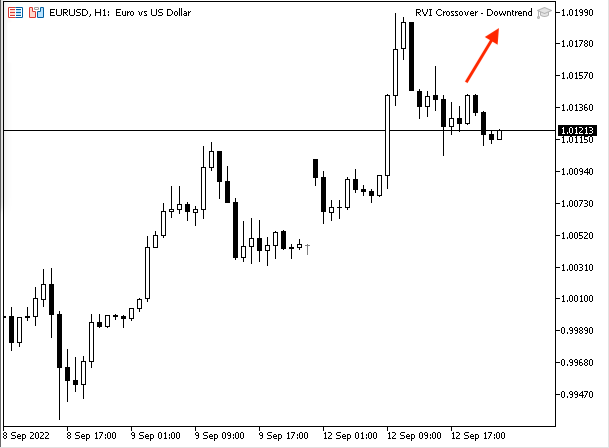Now, we're ready to receive signals based on this strategy and trading system. The following are examples of signals from testing:

In case of short signal: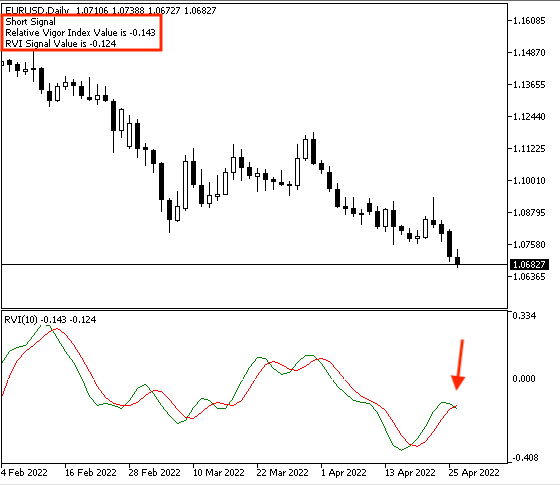As we can see on the chart in the top left corner that we received a signal with the following values:

• Short signal
• Relative Vigor Index value
• RVI signal value

In case of cover: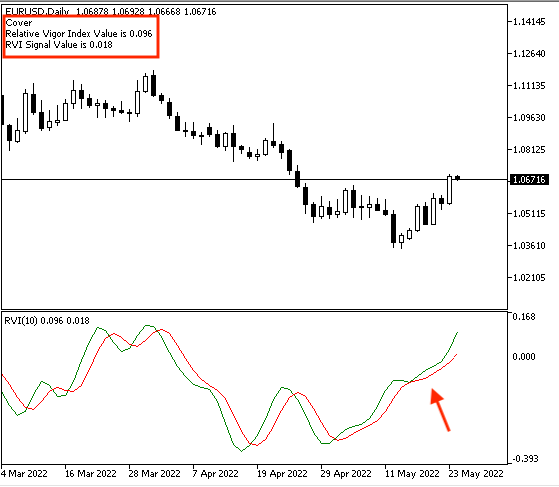As we can see that we have a signal of the following values:

• Cover
• Relative Vigor Index value
• RVI signal value

Now, we have created a trading system based on the strategy of RVI Crossover - Downtrend to generate signals during the downtrend.

• Strategy three: RVI & MA Crossover:

The following is the full code to create a trading system for this trading strategy:

```//+------------------------------------------------------------------+
//|                                           RVI & MA Crossover.mq5 |
//|                                  Copyright 2022, MetaQuotes Ltd. |
//|                                             https://www.mql5.com |
//+------------------------------------------------------------------+
#property version   "1.00"
//+------------------------------------------------------------------+
void OnTick()
{
MqlRates pArray[];
double maArray[];
double rviArray[];
double rviSignalArray[];

int Data=CopyRates(_Symbol,_Period,0,1,pArray);
ArraySetAsSeries(maArray,true);
ArraySetAsSeries(rviArray,true);
ArraySetAsSeries(rviSignalArray,true);

int rviDef = iRVI(_Symbol,_Period,10);
int maDef = iMA(_Symbol,_Period,100,0,MODE_EMA,PRICE_CLOSE);

CopyBuffer(rviDef,0,0,3,rviArray);
CopyBuffer(rviDef,1,0,3,rviSignalArray);

double rviVal = NormalizeDouble(rviArray,3);
double rviSignalVal = NormalizeDouble(rviSignalArray,3);
double maVal = NormalizeDouble(maArray,3);

if(pArray.close>maVal && rviVal>rviSignalVal)
{
"Closing price is ",pArray.close,"\n",
"MA Value is ",maVal,"\n",
"Relative Vigor Index Value is ",rviVal,"\n",
"RVI Signal Value is ",rviSignalVal);
}
if(pArray.close<maVal && rviVal<rviSignalVal)
{
Comment("Sell Signal","\n",
"Closing price is ",pArray.close,"\n",
"MA Value is ",maVal,"\n",
"Relative Vigor Index Value is ",rviVal,"\n",
"RVI Signal Value is ",rviSignalVal);
}

}
//+------------------------------------------------------------------+
```

Differences in this code.

Creating four arrays (pArray, maArray, rviArray, rviSignalArray). We will use the double function the same as what we mentioned except for the pArray, we will use the MqlRates function which stores information about the price, volume, and spread.

```   MqlRates pArray[];
double maArray[];
double rviArray[];
double rviSignalArray[];
```

Setting the AS_SERIES flag to arrays to return a true or false which is a boolean value by using the "ArraySetAsSeries" function. Defining Data by using the "CopyRates" function to get historical data of MqlRates structure and its parameters are:

• symbol_name: to determine the symbol name, we will use _Symbol to be applied to the current symbol.
• timeframe: to determine the period and we will use _Period to be applied to the current period.
• start_pos: to determine the starting position, we will use (0).
• count: to determine the data count to copy, we will use (1).
• rates_array[]: to determine the target array to copy, we will use the pArray.
```   int Data=CopyRates(_Symbol,_Period,0,1,pArray);
ArraySetAsSeries(maArray,true);
ArraySetAsSeries(rviArray,true);
ArraySetAsSeries(rviSignalArray,true);```

Defining RVI by using the iRVI function the same as we mentioned and Moving average by using the iMA function to return the handle of the moving average indicator and its parameters are:

• symbol: to determine the symbol name, we will use (_Symbol)
• period: to determine the period of the timeframe, we will use (_period)
• ma_period: to determine the period of the moving average, we will use 100
• ma_shift: to determine the horizontal shift, we will use (0)
• ma_method: to determine the type of the moving average, we will use exponential MA
• applied_price: to determine the type of price, we will use closing price
```   int rviDef = iRVI(_Symbol,_Period,10);
int maDef = iMA(_Symbol,_Period,100,0,MODE_EMA,PRICE_CLOSE);```

Getting data from the buffer of the RVI and MA indicators by using the "CopyBuffer" function.

```   CopyBuffer(rviDef,0,0,3,rviArray);
CopyBuffer(rviDef,1,0,3,rviSignalArray);

Defining values of RVI, RVI signal, and MA value by using the NormalizeDouble function.

```   double rviVal = NormalizeDouble(rviArray,3);
double rviSignalVal = NormalizeDouble(rviSignalArray,3);
double maVal = NormalizeDouble(maArray,3);```

Conditions of the strategy.

In case of buy signal

```   if(pArray.close>maVal && rviVal>rviSignalVal)
{
"Closing price is ",pArray.close,"\n",
"MA Value is ",maVal,"\n",
"Relative Vigor Index Value is ",rviVal,"\n",
"RVI Signal Value is ",rviSignalVal);
}```

In case of sell signal

```   if(pArray.close<maVal && rviVal<rviSignalVal)
{
Comment("Sell Signal","\n",
"Closing price is ",pArray.close,"\n",
"MA Value is ",maVal,"\n",
"Relative Vigor Index Value is ",rviVal,"\n",
"RVI Signal Value is ",rviSignalVal);
}```

After compiling this code and executing the expert in the terminal, we will find that the expert is attached to the chart the same as the following: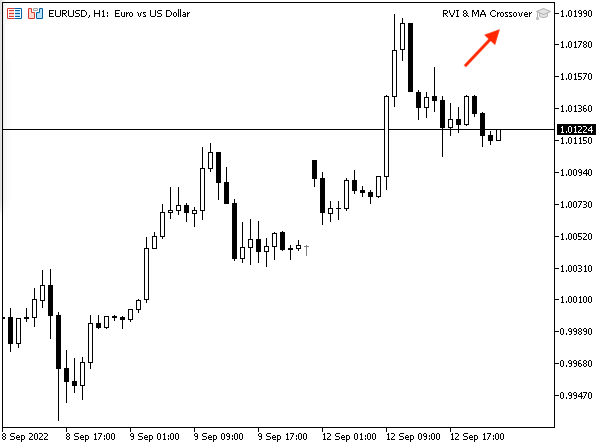Now, we can get generated signals based on this trading system the same as the following examples from testing.

In case of buy signal: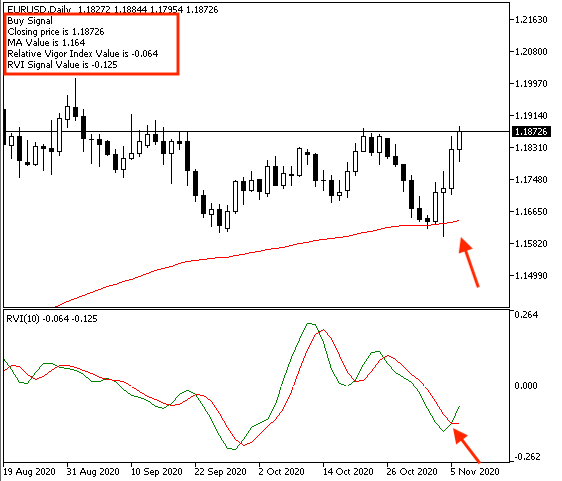As we can see in the previous chart in the top left corner that we have a signal with the following values:

• Closing price
• MA Value
• Relative Vigor Index Value
• RVI Signal Value

In case of sell signal: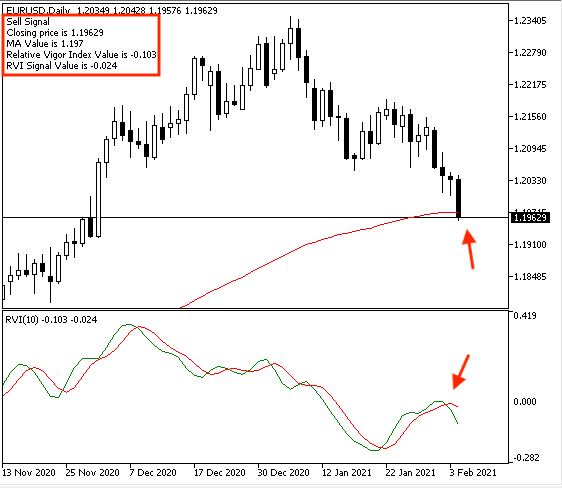We have a signal with the following values:

• Sell Signal
• Closing price
• MA Value
• Relative Vigor Index Value
• RVI Signal Value

Now, we created a trading system for each mentioned strategy to generate an automated signal based on its conditions.

### Conclusion

After what we learned through previous topics in this article, it is supposed that we understood this indicator deeply as we learned about it in detail. We learned what it is, what it measures, and how we can calculate it manually to understand the main concept behind the RVI indicator. We learned how to use it through three simple strategies and they were:

• RVI Crossover - Uptrend: to generate buy and close signals during the uptrend.
• RVI Crossover - Downtrend: to generate short and cover signals during the downtrend.
• RVI & MA Crossover: to generate buy and sell signals based on a specific crossover technique.

We designed a step-by-step blueprint for each mentioned strategy to help us to create trading for them smoothly and easily. We created a trading system for each of mentioned strategies to generate automatic signals after executing them in MetaTrader 5.

I hope that you tried to apply what you learned by yourself to get the maximum benefit from this article and develop your programming skills. I hope also that you found this article useful for you to improve your trading results and get useful insights related to the topic of the article or any related topic. If you want to read more similar articles to learn how to design a trading system based on the most popular technical indicators, you can read my other article in this series about that.

Attached files |
Simple_RVI.mq5 (1.12 KB)

#### Other articles by this author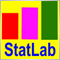The price movement model and its main provisions (Part 2): Probabilistic price field evolution equation and the occurrence of the observed random walk
The article considers the probabilistic price field evolution equation and the upcoming price spike criterion. It also reveals the essence of price values on charts and the mechanism for the occurrence of a random walk of these values.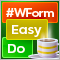DoEasy. Controls (Part 9): Re-arranging WinForms object methods, RadioButton and Button controls
In this article, I will fix the names of WinForms object class methods and create Button and RadioButton WinForms objects.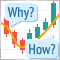Learn how to design a trading system by Awesome Oscillator
In this new article in our series, we will learn about a new technical tool that may be useful in our trading and it is the Awesome Oscillator (AO) indicator. We will learn how to design a trading system by this indicator.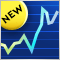CCI indicator. Upgrade and new features
In this article, I will consider the possibility of upgrading the CCI indicator. Besides, I will present a modification of the indicator.